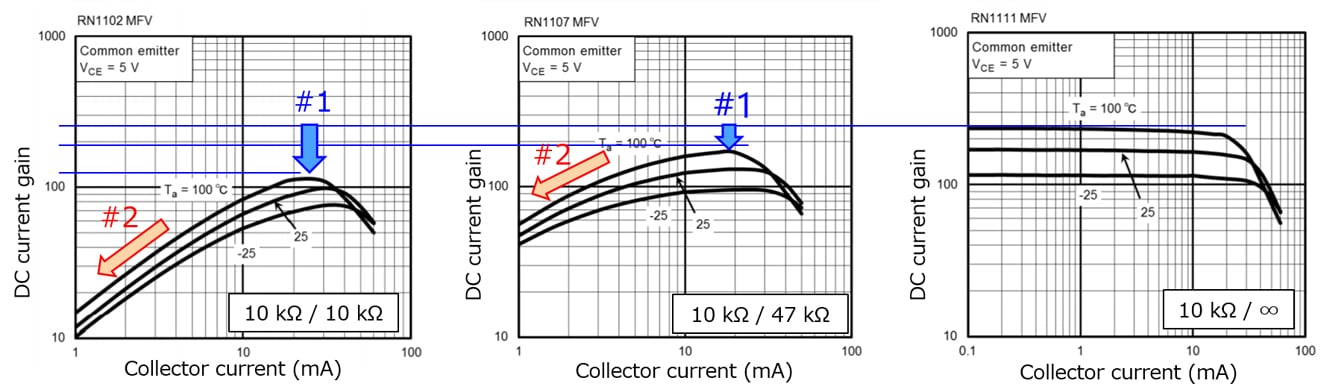# About the hFE of a bias resistor built-in transistor (BRT)

The BRT contains resistors R1 and R2 as shown in Figure 2.*
The definition of the hFE of the BRT is different from that of typical bipolar transistors and is expressed by the following equations:

hFE = IC / IB = IC / ( Ib + IR2 )   ... (1)
IR2 = Vbe / R2 = 0.7** / R2       ... (2)
In the case of typical bipolar transistors or BRTs containing only R1, IB = Ib. Hence, hFE is expressed as:
hFE = IC / Ib            ... (3)

The hFE of the BRT differs from that of typical bipolar transistors in that the denominator of Equation (1) includes IR2.
#1:  Equation (2) indicates that IR2 is inversely proportional to R2. BRTs with a higher R2 value exhibit a higher hFE.
#2:  Ib in the denominator is proportional to IC, but IR2 is a constant value dependent only on R2.
Therefore, hFE decreases in the low collector-current region because of a greater effect of R2.
Obviously, BRTs without R2 and typical bipolar transistors do not exhibit this characteristic.***

*: BRTs without R2 are also available.

**: Vbe changes with collector current (IC). However, since Vbe changes only slightly, it is assumed to be constant at 0.7 V.

***: In the lower collector-current region, hFE might decrease because of the effect of leakage current.Figure 1 Comparison of BRTs with an equal series base resistor (R1) value and different base-emitter resistor (R2) values